1597716000

# Enhance Decision Tree accuracy with Tsallis Entropy

We have been using decision trees for regression and classification problems for good amount of time. In the training process, growth of the tree depends on the split criteria after random selection of samples and features from the training data. We have been using Gini Index or Shannon Entropy as the split criteria across techniques developed around decision tree. And its well accepted decision criteria across time and domain.

Its has been suggested that choosing between Gini Index and Shannon Entropy does not make significant different. In practice we choose Gini Index over Shanon Entropy just to avoid logarithmic computations.

The most methodical part of decision tree is spliting the nodes. We can understand the criticality of the meaurement we choose for the split. Gini Index has worked out for most of the solutions but whats the harm in getting additional few points of accuracy.

The very near by alternative to Gini Index and Shannon Entropy is Tsallis Entropy. Actually Tsallis is not alternative but the parent of Gini and Entropy. Lets see how -

#machine-learning #data-science #entropy #decision-tree #information-theory #deep learning

## Buddha Community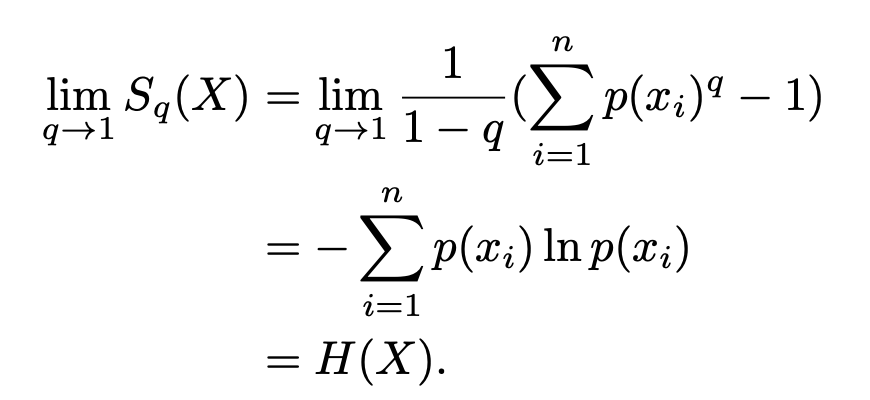1597716000

## Enhance Decision Tree accuracy with Tsallis Entropy

We have been using decision trees for regression and classification problems for good amount of time. In the training process, growth of the tree depends on the split criteria after random selection of samples and features from the training data. We have been using Gini Index or Shannon Entropy as the split criteria across techniques developed around decision tree. And its well accepted decision criteria across time and domain.

Its has been suggested that choosing between Gini Index and Shannon Entropy does not make significant different. In practice we choose Gini Index over Shanon Entropy just to avoid logarithmic computations.

The most methodical part of decision tree is spliting the nodes. We can understand the criticality of the meaurement we choose for the split. Gini Index has worked out for most of the solutions but whats the harm in getting additional few points of accuracy.

The very near by alternative to Gini Index and Shannon Entropy is Tsallis Entropy. Actually Tsallis is not alternative but the parent of Gini and Entropy. Lets see how -

#machine-learning #data-science #entropy #decision-tree #information-theory #deep learning1596286260

## Decision Tree -Classification

Decision Tree is one of the most widely used machine learning algorithm. It is a supervised learning algorithm that can perform both classification and regression operations.

As the name suggest, it uses a tree like structure to make decisions on the given dataset. Each internal node of the tree represent a “decision” taken by the model based on any of our attributes. From this decision, we can seperate classes or predict values.

Let’s look at both classification and regression operations one by one.

# Classification

In Classification, each leaf node of our decision tree represents a **class **based on the decisions we make on attributes at internal nodes.

To understand it more properly let us look at an example. I have used the Iris Flower Dataset from sklearn library. You can refer the complete code on Github — Here.

Image for post

A node’s samples attribute counts how many training instances it applies to. For example, 100 training instances have a petal width ≤ 2.45 cm .

A node’s value attribute tells you how many training instances of each class this node applies to. For example, the bottom-right node applies to 0 Iris-Setosa, 0 Iris- Versicolor, and 43 Iris-Virginica.

And a node’s gini attribute measures its impurity: a node is “pure” (gini=0) if all training instances it applies to belong to the same class. For example, since the depth-1 left node applies only to Iris-Setosa training instances, it is pure and its gini score is 0.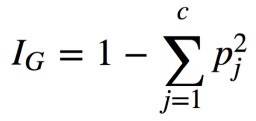Gini Impurity Formula

where, pⱼ is the ratio of instances of class j among all training instances at that node.

Based on the decisions made at each internal node, we can sketch decision boundaries to visualize the model.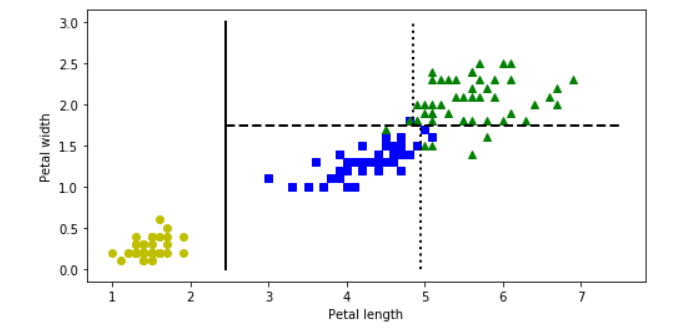But how do we find these boundaries ?

We use Classification And Regression Tree (CART) to find these boundaries.

CART is a simple algorithm that finds an attribute _k _and a threshold _t_ₖat which we get a purest subset. Purest subset means that either of the subsets contain maximum proportion of one particular class. For example, left node at depth-2 has maximum proportion of Iris-Versicolor class i.e 49 of 54. In the _CART cost function, _we split the training set in such a way that we get minimum gini impurity.The CART cost function is given as: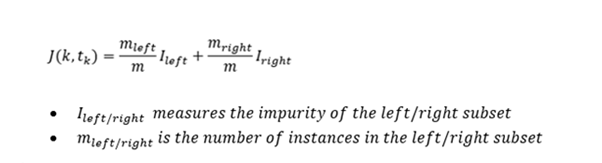After successfully splitting the dataset into two, we repeat the process on either sides of the tree.

We can directly implement Decision tree with the help of Scikit learn library. It has a class called DecisionTreeClassifier which trains the model for us directly and we can adjust the hyperparameters as per our requirements.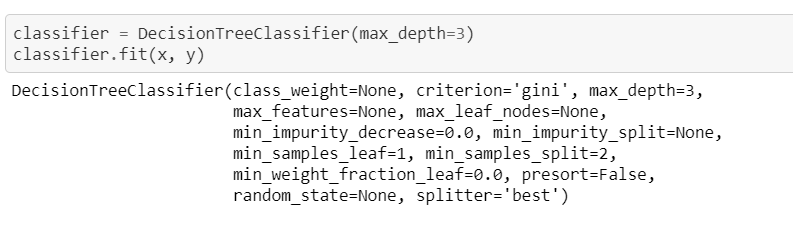#machine-learning #decision-tree #decision-tree-classifier #decision-tree-regressor #deep learning1602997200

## Decision Tree Intuition

Decision Trees are easy & Simple to implement & interpreted. Decision Tree is a diagram (flow) that is used to predict the course of action or a probability. Each branch of the decision tree represents an outcome or decision or a reaction. Decision Trees can be implemented in a variety of situations from personal to complex situations. The sequence of steps will give a better understanding easily.

In Programming, we regularly use If-else conditions, even the Decision Tree working process is similar to an If-else condition.

## Let’s see how a decision Tree Looks like

The below tree shows a simple implementation of different nodes in the decision tree.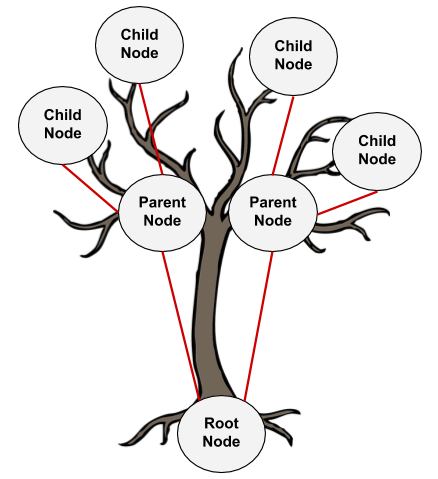1. **Root Node: **Root Node is a top node with the base feature.
2. **Parent Node: **Nodes that get their origin from a root node or this can be represented as a decision node where the decision of Yes/No or True/False or prediction turn happens.
3. **Child Node: **these nodes get their origin from a parent node. If the decision made from a parent node is not satisfactory then these nodes will be created. Until we arrive at the final node where we have pure domination in a class (Yes/No) means that until we arrive at the leaf node.
4. **Leaf Node: **Can also be called a terminal Node or a final decision node where we will conclude.

#gini-index #entropy #decision-tree #decision-tree-classifier #machine-learning1596285180

## Decision Trees Classifier

Both of `Regression Trees` and `Classification Trees` are a part of `CART (Classification And Regression Tree) Algorithm`. As we mentioned in Regression Trees article, tree is composed of 3-major parts; root-node, decision-node and terminal/leaf-node.

The criteria used here in node splitting differs from that being used in Regression Trees. As before we will run our example and then learn how the model is being trained.

There are three commonly measures are used in the attribute selection `Gini impurity` measure, is the one used by `CART` classifier. For more information on these, see Wikipedia.

# Data set being used is `iris` data set

``````import numpy as np
import pandas as pd
from sklearn.tree import DecisionTreeClassifier
from sklearn.tree import export_graphviz
from six import StringIO
from IPython.display import Image
# pip/conda install pydotplus
import pydotplus
from sklearn import datasets
xList = iris.data  # Data will be loaded as an array
labels = iris.target
dataset = pd.DataFrame(data=xList,
columns=iris.feature_names)
dataset['target'] = labels
targetNames = iris.target_names
print(targetNames)
print(dataset)
``````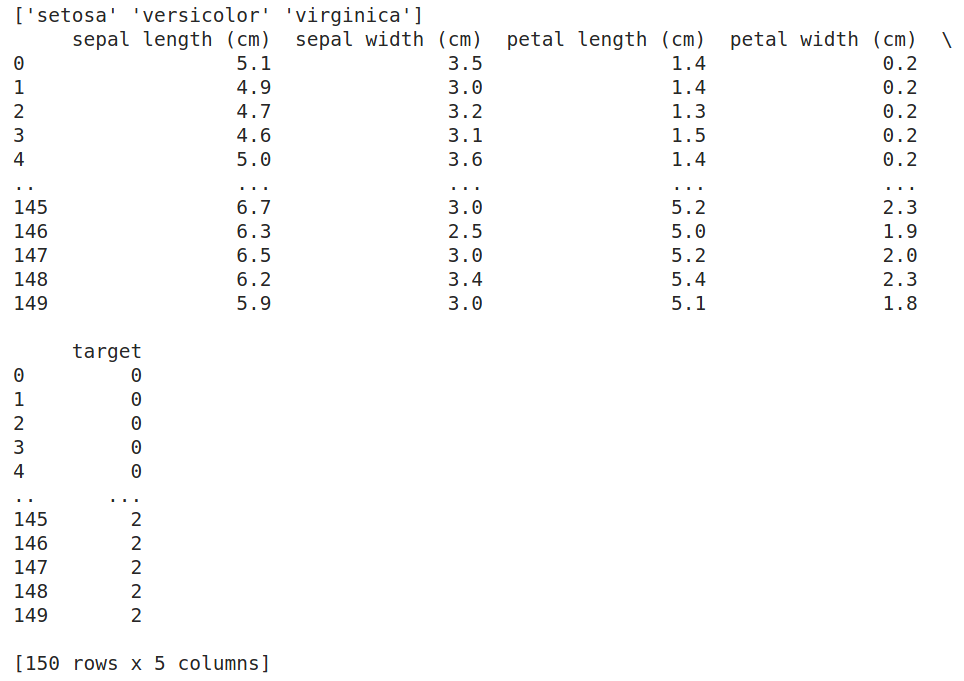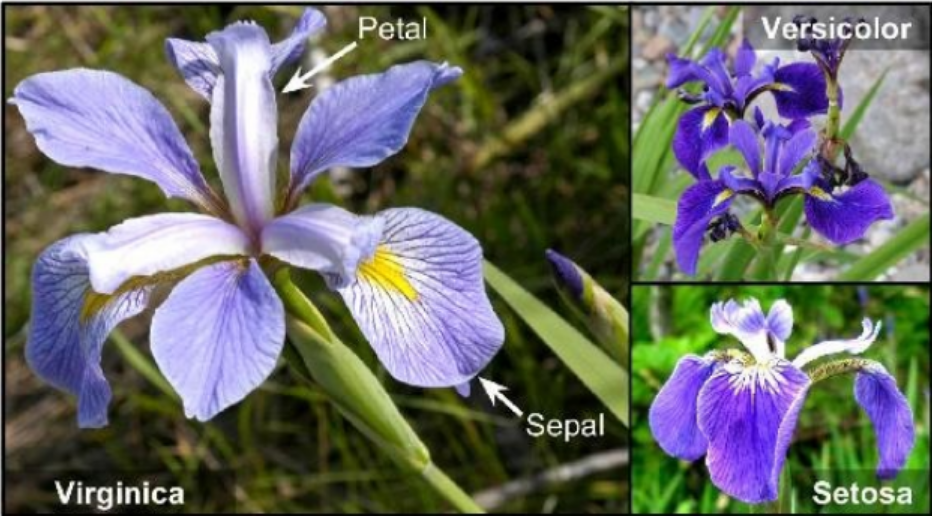Iris Flowers

# How a Binary Decision Tree Generates Predictions

When an observation or row is passed to a non-terminal node, the row answers the node’s question. If it answers yes, the row of attributes is passed to the leaf node below and to the left of the current node. If the row answers no, the row of attributes is passed to the leaf node below and to the right of the current node. The process continues recursively until the row arrives at a terminal (that is, leaf) node where a prediction value is assigned to the row. The value assigned by the leaf node is the mean of the outcomes of the all the training observations that wound up in the leaf node.

# How to split the nodes

Classification trees split a node into two sub-nodes. Splitting into sub-nodes will increase the homogeneity of resultant sub-nodes. In other words, we can say that the purity of the node increases with respect to the target variable. The decision tree splits the nodes on all available variables and then selects the split which results in most homogeneous/pure sub-nodes.

There are major measures being used to determine which attribute/feature is used for splitting and which value within this attribute we will start with. Some of these measures are:

• Gini index (Default in SciKit Learn Classifier).
• Entropy.
• Information gain.

We will start with `Gini index` measure and try to understand it

# Gini Index

Gini index is an impurity measure used to evaluate splits in the dataset. It is calculated by getting the sum of the squared probabilities of each class (target-class) within a certain attribute/feature then benig subtacted from one.

#machine-learning #mls #decision-tree #decision-tree-classifier #classification #deep learning1592847556

## Binary Decision Trees

Binary Decision Trees. Binary Decision Trees
Binary decision trees is a supervised machine-learning technique operates by subjecting attributes to a series of binary (yes/no) decisions. Each decision leads to one of two possibilities. Each decision leads to another decision or it leads to prediction.

#decision-tree-regressor #decision-tree #artificial-intelligence #mls #machine-learning #programming